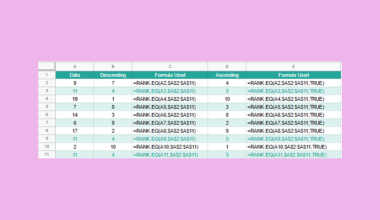# How To Use IMEXP Function in Google Sheets

The IMEXP function in Google Sheets is useful when you need to raise Euler’s number, also known as e,  to a complex power.

Euler’s number (~2.718) has special properties when raised by an exponent. Using the IMEXP function allows you to raise e to a complex number of the form a+bi.

The rules for using the `IMEXP` function in Google Sheets are as follows:

• The function requires just one argument. The user must provide the complex number that we will raise e to.
• The function then outputs the result of e raised to that number.
• The formula for raising e to a complex power is as follows: IMEXP(x+yi) = excos(y) + iexsin(y)

Let’s take a look at a quick example!

In this example, we have a spreadsheet with a sample of complex numbers. We would like to return the value of e raised to the power of n, where n is a complex number in our sample dataset. Using the `IMEXP` function, we can easily return the value we are looking for.

Let’s learn how to write the `IMEXP` function ourselves in Google Sheets and later test out the function to solve the example stated earlier.

## The Anatomy of the IMEXP Function

So the syntax (the way we write) the `IMEXP` function is as follows:

```=IMEXP(exponent)
```

Let’s dissect this thing and understand what each of these terms means:

• = the equal sign is how we start any function in Google Sheets.
• IMEXP() is our `IMEXP` function. It computes Euler’s number raised to a given complex power.
• exponent refers to our complex power, given in the form a+bi.

## A Real Example of Using IMEXP Function

Let’s look at an example of the `IMEXP` function being used in a Google Sheet spreadsheet.

In the table below, we have a sample set of complex numbers in Column A. Using the `IMEXP` function, we’re able to retrieve the values of e raised to each complex number.To get the values in Column B, we just need to use the following formula:

```=IMEXP(A2)
```

You can make a copy of the spreadsheet above using the link I have attached below.

In another example, we’ve used the same dataset but have also extracted the real and imaginary coefficients.This is possible thanks to the `IMREAL` and `IMAGINARY` functions in Google Sheets, which allow us to retrieve the real and imaginary coefficients of our `IMEXP` output.

If you’re ready to try out the `IMEXP` function in Google Sheets, let’s begin writing it ourselves!

## How to Use IMEXP Function in Google Sheets

1. Our guide starts by having an idea of what our inputs are. In the table below, we have our complex numbers placed in Column A. To start using the `IMEXP` function, we must first select the cell we would like to contain our output. In this case, we’ll start with cell B2.2. Next, we just simply type the equal sign ‘=‘ to begin the function, followed by ‘IMEXP(‘.
3. As seen below, a tooltip box appears with info on the `IMEXP` function. We can click on the arrow on the top-right-hand corner of the box to minimize it if necessary.4. Next, we need to provide the cell which contains the complex number we want to use.
Afterward, simply hit Enter on your keyboard to let the function evaluate.5. Finally, we can drag down the formula to evaluate our formula for the rest of the dataset.1. Why does my formula output a #NUM! error?
A #NUM! error indicates that the complex number provided is invalid. Make sure that the complex number follows the form a+bi.
2. How do I make sure that my number argument is always a valid complex number?
To make sure that the argument for the `IMEXP` function is always valid, we can use the COMPLEX function to generate our complex numbers. For example, we can use the formula =COMPLEX(4, 2, ”i”) to create the complex number ‘4 + 2i’.

That’s all you need to know on how to use the `IMEXP` function in Google Sheets. This step-by-step guide shows how simple it is to raise e to a certain complex power.

You can now use the `IMEXP` function in Google Sheets with various other formulas available in Google Sheets to create great worksheets that make your work and life easier for you.

Don’t miss out on more Google Sheets knowledge by staying notified of new guides by subscribing to our newsletter!Our goal this year is to create lots of rich, bite-sized tutorials for Google Sheets users like you. If you liked this one, you'd love what we are working on! Readers receive ✨ early access ✨ to new content.

##### You May Also Like## How to Quickly Split Text in Google Sheets

In this article, we’ll learn how to quickly split text in Google Sheets. We’ll see more than one…## How to Convert Month Name to Number in Google Sheets

Knowing how to convert month name to number in Google Sheets is useful if you have your month…## How to Use RANK.EQ Function in Google Sheets

The RANK.EQ function in Google Sheets is used to determine the rank of a specific value in a…## How To Use NOW Function In Google Sheets

The NOW function in Google Sheets is useful if you want to return the current date and time…